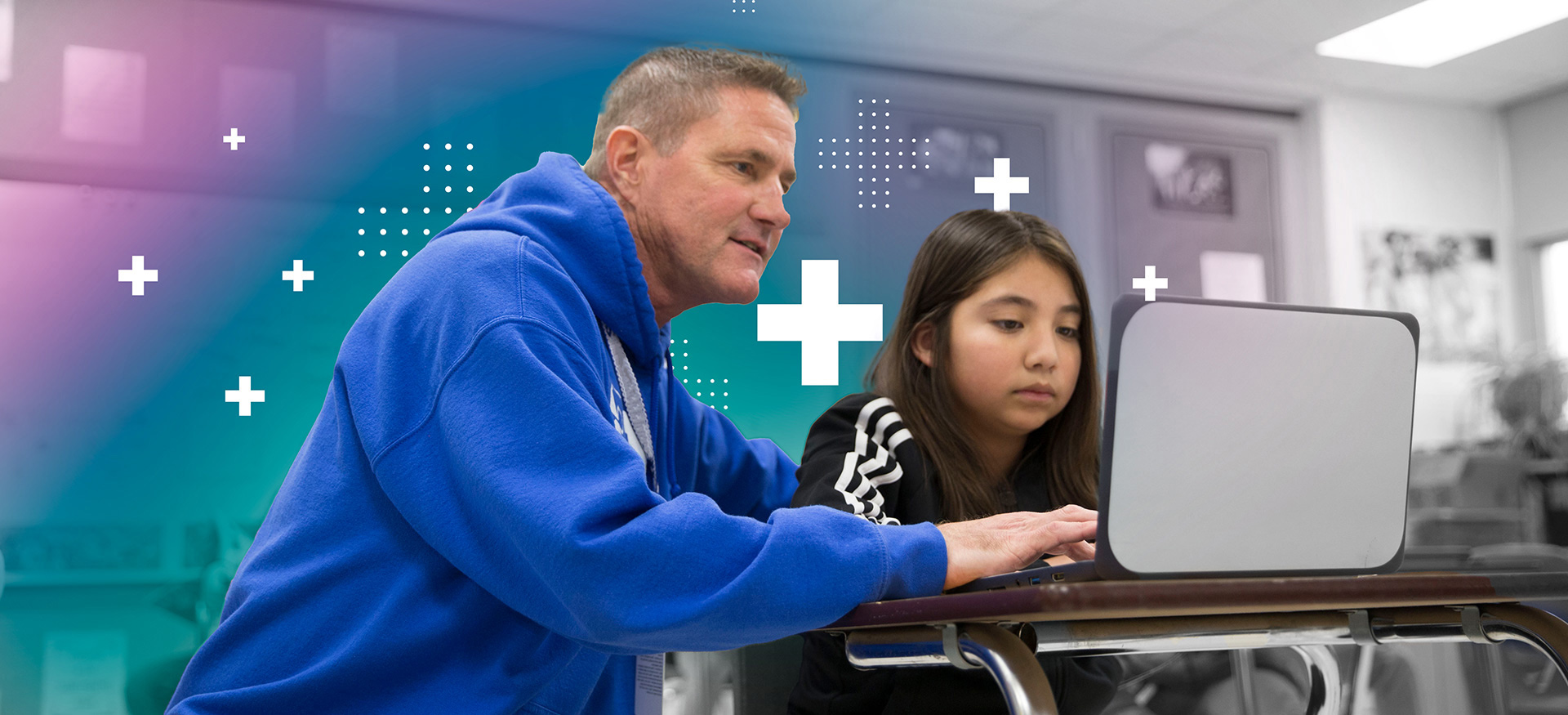# Grades 3–5: Developing Deep Conceptual Understanding of Fractions

Professional Learning Workshop## Discover the mathematical concepts underlying fractions to support student understanding.

As students progress and build their mathematical understanding, flexibility and accuracy with fractions are key elements for determining student success. Support all students in building their understanding of a fraction as a single number and conceptualizing fractions as part of the number system.

## Explore a key question during each segment of learning

#### How can we expand our understanding of a fraction as a single number to include partitioning (cutting) and iterating (copying)?

• Understand fraction constructs to include partitioning and iterating.

#### How can we apply an expanded understanding of fractions to make sense of and solve problems?

• Explain how partitioning and iterating enhance students’ relational understanding of fractions.

#### How can we apply our expanded understanding of fractions to make sense of equivalent fractions and to compare fractions?

• Use different strategies to make sense of equivalent fractions and to compare fractions.
• Understand how to use a number line to reinforce the idea of a fraction as a single number.

#### What are common misconceptions about operations with fractions?

• Investigate misconceptions about operations with fractions.

#### How can we make sense of operations with fractions?

• Practice and make sense of operations with fractions.

#### How can formative assessment strategies be used to equitably promote student success with fractions?

• Experience formative assessment strategies that equitably promote student success with fraction operations.

#### What are the five practices for orchestrating mathematical discourse?

• Develop a cursory understanding of the five practices as a framework for mathematical discourse.### Designed with you in mind

This offering is divided into two 3-hour parts. It is recommended that the parts be completed in order, within a reasonable time frame. Your NWEA representative will help determine the best on-site or virtual delivery option to meet your needs.

#### On-site learning

Face-to-face session with a consultant

#### Virtual learning

Live instruction through our online portal